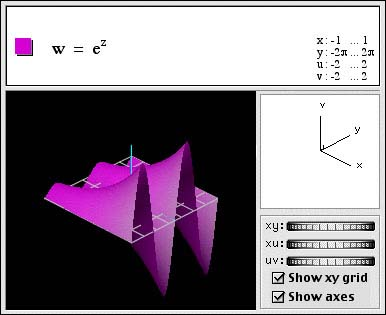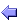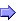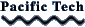### Functions of a complex variable in four dimensions

A function of a complex variable represents a 2-manifold embedded in four dimensions. Here, the white grid is the z = x + iy complex plane. The function w = ez, or u + iv = ex + iy is projected from four-dimensional (x,y,u,v) space to the screen, in a similar same way a 3D image is projected onto a 2D picture. This projection shows the imaginary part of ex + iy = ex(cos y + i sin y). One can see both the sinusoidal variation with y and the exponential variation with x in this view.